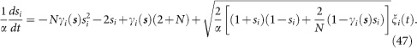## Introduction

With the exception of a small class of problems that are solvable analytically, most quantum many-body problems can only be examined using numerical means, for which exact simulations scale exponentially with the problem size. Approximate methods such as quantum Monte Carlo and Density Matrix Renomalization Group (DMRG) give accurate results for certain cases but no general algorithm exists that can be applied to an arbitrary system. In the field of quantum information technology, quantum simulation has gathered a large amount of attention as an alternative means to study such quantum many-body problems. A quantum simulator is a device where a quantum many-body problem of interest is artificially created in the lab, such that its properties can be controlled and measured directly1. By directly using quantum mechanics in the simulation, there is no exponential overhead in keeping track of the number of states in the Hilbert space of the problem. This is in the spirit of Feynman's original motivations for quantum computing2, where quantum mechanics, rather than classical mechanics, is used to simulate quantum many-body problems.

Given this general approach to quantum many-body problems, the question of whether a quantum simulation approach can be applied to a general Ising model becomes an important question. The Ising model problem consists of finding the lowest energy state of the Hamiltonian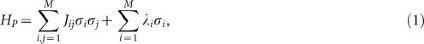where Jij is a real symmetric matrix that specifies the connections between the sites i, j and σi = ±1 is a spin variable. The task is then to find the minimal energy spin configuration {σi} of the Hamiltonian (1). The problem of finding the solution of the Hamiltonian (1) is in fact known to be NP-complete, since it can be trivially mapped onto the MAX-CUT problem3. Furthermore, it can in principle encode an arbitrary NP-complete problem by a polynomial time mapping procedure4, thus the potential application of a reliable method of solving (1) is extremely broad. Although (1) is itself a classical Hamiltonian since it does not contain any non-commuting operators, as with quantum annealing where the Hamiltonian is supplemented with an additional transverse field5, quantum “tricks” may be used to speed up the solution of the ground state beyond classical methods.

In a previous work we investigated a computational device which finds the solution of an Ising model by a set of interconnected Bose-Einstein condensates6,7. In the approach of Ref. 6, each spin was replaced by a system of N bosons which can take one of two states. By implementing an analogous Hamiltonian to (1) and cooling the system down into the ground state, it was shown that the solution of the original Ising model problem could be found. There is a speedup compared to simply implementing (1) using single spins, because of the presence of bosonic final state stimulation within each bosonic spin. This resulted in finding the solution of (1) with a speedup of approximately N. The attractive feature of the proposal in Ref. 6 is that the computation is done simply by implementing a static Hamiltonian, so that no complicated gate sequence needs to be employed in order to find the ground state solution. Effectively, the dissipative process itself performs the computation itself and therefore can also be considered to be a reservoir engineering approach to quantum computation. Related proposals were offered in Refs. 8,9,10, where instead of BECs, photons were used.

In this paper, we analyze the proposal in Ref. 6 from the point of view of neural networks, specifically the stochastic continuous Hopfield model. Recasting the proposal in this form allows for a clearer analysis of the properties of the device, where standard results can be carried over to the BEC case. It clarifies the origin of the ~ N speedup of the device, which was established via a numerical approach in Ref. 6. We find that the ~ N speedup originates from each element of the Hopfield network being accelerated due to bosonic stimulation and thermal fluctuations provide the stochastic aspect to the Hopfield network. We then consider some simple applications of the BEC network for neural networking tasks.

## Results

### Bose-Einstein condensate networks

We first give a brief description of the proposal of Ref. 6. In order to solve (1) we consider a system such as that shown in Figure 1. Each spin σi in HP is associated with a trapping site containing N bosonic particles. The bosons can occupy one of two spin states, which we label by σ = ±1. The set of possible states can then be written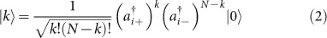where a is the annihilation operator for spin σ on site i. A concrete example of the two levels for the case of atomic BECs can be found in Fig. 6(a) of Ref. 11. On each site i we may define a total pseudospin (which we call spin henceforth for brevity) operator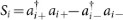taking eigenvalues Si|k〉 = (−N + 2k)|k〉. The sites are controlled such that the system follows the bosonic Ising Hamiltonian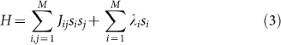where Jij is the same matrix as in HP which specifies the computational problem and si = Si/N are normalized spin variables.

The ground state of (3) can be used to infer the ground state of (1). This follows from the fact that for extremal states si = ±1, the same structure of the Hamiltonian gives the same ordering of energy states. However, unlike the discrete σi variables, si take a large number of values between −1 and 1 and thus there are many more states present in (3) in comparison to (1). One may then worry that some of these states are in fact lower than the ground state. As discussed in Ref. 6, it can be shown that such states can never be lower in energy than the extremal states and are at worst degenerate with the ground state configuration of (1). Thus one may always deduce a ground state of (1) by making the assignment σi = si/|si|, provided si is a ground state configuration.

One possible method of creating the interactions experimentally in (3) is via a measurement-feedback approach. In this approach, the total spin si on each site is continuously measured and an appropriate control signal is continuously fed back into the system by applying a local dc field on another site. Given a measurement result of {sj(t)} across the spins, a local field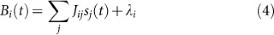is applied on site i. Since the Zeeman energy due to this field is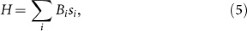a simple substitution yields (3).

Starting from a random spin configuration {sj(t)}, the system is cooled assuming that the ambient temperature T is fixed. The procedure is essentially identical to a simulated annealing procedure, the sole difference being the use of the bosonic Ising model. By varying the temperature during the cooling process such that it is time dependent T(t), a strategy similar to thermal annealing may be performed, in order to escape being trapped in local minima. In practice, instead of varying the temperature, varying the overall magnitude of (3) by adjusting the strength of the magnetic field (4) is equivalent. By taking advantage of the bosonic amplification of the cooling process, it was found in Ref. 6 that an approximate factor of N was found in the cooling process.

The time evolution is modeled by an extension of the method presented by Glauber to bosons18. Given the M site Hamiltonian (3), the states are labeled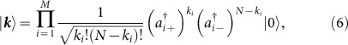where the ki range from 0 to N,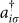is the creation operator for a boson on site i in the state σ and we have defined the vector k = (k1, k2, …, kM). The approach as described in Ref. 6 is to assign a probability pk of occupation of each configuration labeled by |k〉. The system then evolves between the states |k〉 → |k′〉 by a probabilistic process, characterized by transition weight factors w. The weight factors are chosen such that in the long time limit, the states k obey a thermal equilibrium statistics.

Given an initial probability distribution set by the initial conditions, pk then evolves according to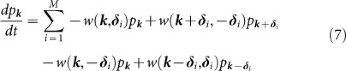where δi is a vector of length M with its ith element equal to one and zero otherwise. The w(k, δi) is a weight factor representing the transition |k〉 → |k + δi〉, containing both the bosonic final state stimulation factor and a coefficient to ensure that the system evolves to the correct thermal equilibrium distribution. We have restricted the transitions to first order transitions in (7) for simplicity. The final state stimulation factor can be calculated by assuming a Hamiltonian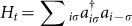and calculating the transition rate according to Fermi's golden rule (up to a constant)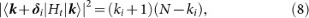At thermal equilibrium, the transition rates are equal between the states |k〉 ↔ |k + δi〉, which ensures that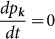. The final state stimulation factors cancel for such pairs and we have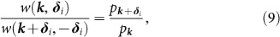and similarly for δi → −δi. From the probability distribution at thermal equilibrium we can calculate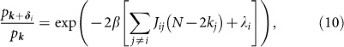where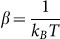. This gives the coefficients as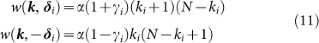where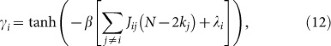α is a constant determining the overall transition time scale. α is set to 1 for all numerical calculations.

### Equivalence to the stochastic continuous Hopfield model

We now show that the scheme detailed in the previous section is formally equivalent to the stochastic continuous Hopfield model. After defining the Hopfield model we show the equivalences between the two systems and derive the evolution equations for the BEC network in the context of the Hopfield model.

#### Definition of the Hopfield model

The Hopfield model is an asynchronous model of an artificial neural network12,13, where the each unit of the network changes state at random times, such that no two units change simultaneously. The Hopfield model is in the class of recurrent neural networks, where the output of the network is fed back into itself, so that given a particular initial configuration, the system undergoes a sequence of changes in configuration of the units until steady state is achieved.

In the standard (discrete, deterministic) Hopfield model, each unit takes the value σi = ±1. The units are updated according to the rule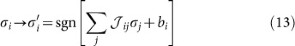where sgn(x) = x/|x| denotes the sign function,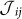is a symmetric matrix with zero diagonal elements, bi is the threshold of unit i. The units are updated to their new values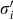at random. Whether a given state is stable with respect to the updates can be determined by the Lyapunov (energy) function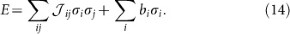The update sequence proceeds until a local minima with respect to the Lyapunov function is reached. From a physics perspective, the transition rule (13) can be viewed as a cooling process at zero temperature, where given an initial high energy spin configuration, the spins cool one by one randomly into a low energy state. It is thus equivalent to the discrete Ising model problem (1) up to a constant energy factor originating from the diagonal elements of Jij, which play no part in the dynamics of the problem.

The model can be extended to one where continuous variables xi [−1, 1] are used in place of the discrete ones σi. Such continuous Hopfield networks have similar properties to the discrete version in terms of the configuration of stable states14. The way the model is usually defined is in terms of an electric circuit model (see for e.g. Ref. 13). On a single unit i, the time evolution of the circuit obeys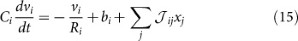where Ci is the capacitance, Ri is the resistance, vi is the voltage and xi is the output of the circuit after operation of the nonlinear amplifier (or activation function). The activation function restricts the output to a limited region such that output is always in the range [−1, 1]. A typical choice is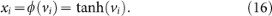The corresponding energy function for the dynamics is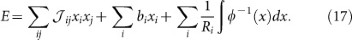From the energy function it may then be shown that the system is guaranteed to converge to a local minima of the energy. The last term in (17) gives a modification of the energy landscape which shifts the position of the minima away from the extrema x = ±1. For a sufficiently high-gain amplifier (corresponding to a modification of the activation function to ϕ(vi) → ϕ(Gvi) with G > 1), this term is known to have a negligible effect on the overall dynamics14. Due to the energy structure of the continuous model being equivalent to the discrete model13, a solution to the continuous model then gives a one-to-one correspondence to the discrete model.

#### Equations of motion on a single site

In order to see the equivalence with the Hopfield model, let us first examine the dynamics on a single site and set M = 1. In this case the probability distribution of the states evolve as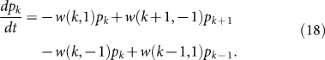where in this case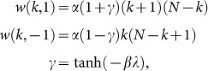since there is only one site. Multiplying the whole equation by k and summing over k given an equation for the mean value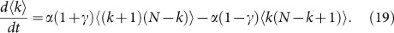Making the approximation that 〈k2〉 ≈ 〈k2 and changing variables to S = −N + 2k the equation can be recast into the form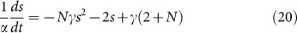where we have used the normalized variable s = 〈S〉/N. This approximation is accurate under physically relevant probability distributions generated by (18). For example, a uniform distribution pk = 1/N gives 〈k2N2/4, in comparison to the exact result 〈k2〉 ≈ N2/3. The approximation improves as the distribution becomes more peaked. An explicit solution for this may be found to be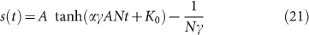where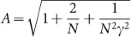is a constant that is order unity at low temperatures such that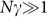. K0 is fixed by the initial conditions, for which we typically assume s(t = 0) = 0. We see that the spin approaches its steady state value with a timescale ~ 1/α|γ|N. At zero temperature where γ = ±1 (depending upon the direction of the applied external field), the time scale is enhanced by a factor of N.

The physical origin of the speedup is due to bosonic final state stimulation. When N indistinguishable particles occupy a quantum state, the rate of population transfer is enhanced by a factor of ~ N. This effect is most familiar from the theory of lasing, where photon emission occurs preferentially into the lasing mode15. In our case, the cooling rate of the bosons on a single site is accelerated by a factor of N, due to the bosonic factors (8) in the transition rates (11). This accelerated cooling is what provides the speedup of the network as a whole6.

Let us compare this to the Hopfield network for a single site. In this case the equation of motion reads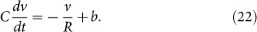Changing variables to the output of the nonlinear amplifier, we obtain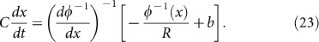This is the counterpart of the equation (20) for the Hopfield case. The solution of this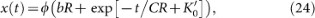where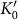is set by the initial conditions.

Writing the equations in this form makes the correspondence between the BEC system and the Hopfield network clear, which we summarize in Table I. The output x of the Hopfield network corresponds to the normalized spin variable s. The magnetic field B applied on each site for the BEC system then corresponds to the voltage on each Hopfield unit before the non-linear amplifier. The steady state values are determined by setting the right hand side of (20) and (23) to zero. The value of the steady state depends upon the ratio of the field (λ or b) applied and the temperature kBT = 1/β or the conductance 1/R respectively. The overall time constant is controlled by 1/α or C in each case. In the Hopfield network, there is obviously no bosonic final state stimulation, so the speedup proportional to N is absent. While the exact equation of motion for the two cases (20) and (23) differ in their details, it is clear that the qualitatively there is a similar structure and behavior to the dynamics. While in the Hopfield network, the overall time constant is determined by the capacitance of the circuit, the fundamental timescale of the BEC network is determined by the cooling rate of the bosons on each site.

The analogue of the activation function ϕ(v) may be derived as follows. Considering the activation function as a rule that converts the internal magnetic field B to the spin variable s, we may derive the average spin at thermal equilibrium using the Boltzmann distribution taking into account of bosonic statistics. From the partition function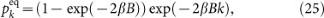and thereforewhere z = here. The function above has a dependence that has similar behavior to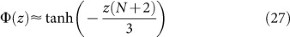which makes it clear that it plays a similar role to that of the activation function ϕ in the Hopfield network.

#### Equations of motion for interconnected BECs

We may now generalize to the multi-site case. Multiplying (7) by ki and summing over all k gives the equations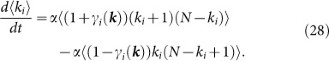where we have written γi → γi(k) to remind ourselves that this is not a constant in this case. Making the approximation that 〈km〉 ≈ 〈km and making the change of variables to si = 〈Si〉/N, we obtain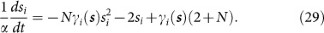The sole difference to the single site case here is that the equilibrium values of si are now dependent on the spins of all the other sites s. The dynamics on each site is the same as the single site case and thus evolves in time as (21), considering the other spins to be approximately fixed. This basic structure is precisely the same dynamics that determine the equation of motion of the Hopfield network (15).

Although it is not possible to solve the set of equations (29) analytically, we may see in this formulation why the whole system should have a speedup of ~ N, as found in Ref. 6. Considering an asynchronous update procedure (in fact this is exactly what was performed to simulate the dynamics of the system in the Monte Carlo procedure7), all but one “active” site is fixed in spins. The active site then evolves in time according to the evolution of (21). This has a speedup of ~ N|γ| in the evolution of the spin, thus to make an incremental change δs in the active spin takes a time reduced by N|γ| compared to the N = 1 case. The spin is then fixed and then another site is chosen at random and this is updated. Since each step takes a reduced time of N|γ|, the whole evolution proceeds at an accelerated rate. For sufficiently low temperatures, γ ≈ ±1 and therefore the speedup is approximately ~ N.

#### Stochastic Hopfield network

Up to this point, the equations of motion (29) have been entirely deterministic. The role of the temperature was to merely shift the equilibrium values of the spins, as determined by (26) and did not contribute to any stochastic evolution of the system. In the BEC network there are thermal fluctuations which cause the system to behave in a random manner. Therefore in order to fully capture the dynamics of the BEC network we must include the contribution of the random thermal process. Such stochastic versions of Hopfield networks and their generalization to the Boltzmann machine (by having additional hidden units) are defined by modifying the update rule (13) to include probabilistic effects. Considering the discrete Hopfield model first, the algorithm consists of selecting a particular spin σi and making an update according to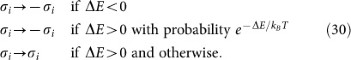ΔE is the energy difference between the state with the flipped spin and no flipped spin16.

The evolution equations (7) for the BEC network can be converted into a set of stochastic update rules which give the same time dependence when an ensemble average is taken. The stochastic formulation also allows for a convenient method of numerically simulating the system, which was discussed in detail in Ref. 7. We briefly describe the procedure as applied to the current formulation of the BEC network. The simulation is started from a random initial value of k = (k1, k2, …, kM) in (7) and we update the system by repeating the stochastic transition process following the kinetic Monte Carlo method17. In each update we calculate the transition weight w(k, δi) in (7) for all the possible transitions. The transition is then made with a probability in proportion to the transition weight w(k, δi). The time increment is then calculated according to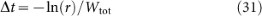where r (0, 1] is a randomly generated number and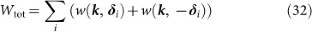This procedure is repeated for many trajectories so that ensemble averages of quantities such as the average spin can be taken.

This procedure produces exactly the same time dynamics as (7), hence for quantities such as the equilibration time this procedure must be followed. However, if only the behavior at equilibrium is required, the update procedure can be replaced by the Metropolis algorithm. The update procedure is then as follows. Start from a random initial value of k = (k1, k2, …, kM). Then make an update according towhere the energy difference in this case is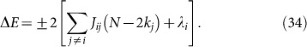The exponential factor is precisely the same as that determining the weight factors in (10). The only difference in this case is that the bosonic stimulation factors are not present in this case, which are important only for determining the transition rates and not the equilibrium values. The above Metropolis transition rule (10) is identical to the stochastic Hopfield network, up to the difference that each site contains energy levels between ki = 0, …, N. It is therefore evident in this context that the two systems are equivalent in their dynamics.

We may also derive the equivalent stochastic differential equations for the average spin by adding noise terms to (29) (see Methods)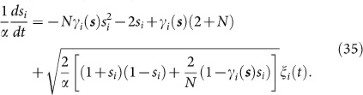The above equation is identical to (29) up to the Gaussian noise term. This allows for the system to escape local minima in the energy landscape. The steady state evolutions then approach the correct thermal equilibrium averages as defined by the Boltzmann distribution. Combined with an annealing schedule, this may be used to find the ground state of the Ising Hamiltonian. As found previously, due to the factors of N in (35) originating from bosonically enhanced cooling, the annealing rates may be made N times faster, allowing for an accelerated method of finding the solution to Ising model problems, as claimed in Ref. 6.

### Learning and pattern completion

Our discussion to this point has focused on the problem of finding the ground state given a particular problem instance encoded by the Jij parameters. This may be given for example by translating a MAX-CUT problem into the corresponding Jij matrix. The task is then to try and find the optimal spin configuration for this problem instance. However, given the correspondence of the BEC network to the continuous Hopfield model, it is clear that there should be other applications beyond this problem solving scenario.

One common application of neural networks is learning and memory retrieval. In this application the network is initially exposed to several patterns that the user would like to retrieve later. In this step the Jij matrix is modified according to a prescribed learning algorithm. Thus in contrast to the previous application Jij is the quantity to be determined in the learning step. Once the learning process is complete, the network is put in retrieval mode and the Jij parameters are fixed. The network now operates in the same way as the problem solving scenario considered previously. Given an initial spin configuration that is similar to the stored configurations, the network then retrieves the stored patterns, demonstrating pattern completion.

In this section, we discuss the application of learning and memory retrieval in BEC networks. The first step of learning can be straightforwardly adapted from standard methods13. To illustrate the technique, we consider the Hebbian learning, one of the simplest unsupervised learning algorithms. The performance of the pattern completion process is then assessed by examining the time taken to retrieve the patterns. This serves to both illustrate the equivalence of the BEC network to the continuous Hopfield model and show the potential benefits of using many bosons in the system.

#### Learning

The simplest example of unsupervised learning is the Hebbian learning rule. Using the associations in Table I it is straightforward to write down the corresponding rule in the case of the BEC network. We follow the presentation given in Ref. 13 (sec. 2.5) for the case of continuous activation functions, since in the BEC system the measured spin is a continuous quantity. We assume that the BEC network starts with the system shown in Figure 1 with the Ising matrix set to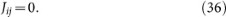The learning algorithm then proceeds as follows. We apply various magnetic field configurations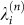where n labels the various pattern configurations that the network is exposed to during the learning process. Starting with the first field configuration n = 1, we apply this field and wait until the spins reach their equilibrium value, which will be given (for the first iteration)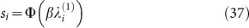We then update the Ising matrix according to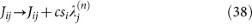where c is the learning constant which determines the speed of the learning process. We then make subsequent applications of the field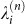, measure the field si in each case, then make the replacement (38). For n ≥ 2, the spins do not simply take the values of (37) since Jij will in general be non-zero. The process is continued until the learning examples are exhausted, or the same set can be recycled. Other learning algorithms may be derived in a similar way using the associations in Table I.

We now discuss in more detail the experimental configuration to realize the Hebbian learning rule for the BEC network. In the configuration of Figure 1, we assume that the Jij matrices can be changed as desired, i.e. they are computer controlled. We also assume that the spins si can be read out in addition to the existing feedback circuit. The experimental procedure then is as follows. 1) Set Jij = 0; 2) Apply the field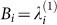to the sites and wait for equilibration; 3) Read the spins si and apply the update rule (38); 4) Apply the field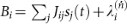and wait for equilibration; 5) Go to step 3 until the patterns are exhausted. This builds up the Jij matrix which can be used in the next step for pattern recognition.

#### Pattern completion

As a simple example of the use of the BEC network for pattern completion, we test the set of equations given in (29) using an Ising matrix trained using the Hebbian algorithm of the previous section. We numerically evolve a set of 16 × 16 equations forming a two dimensional grid at zero temperature from the initial conditions as shown in Figure 2. In Figures 2a and 2b we start from fragments of the learned patterns while in Figure 2c we start from a randomly chosen spin configuration. We see that in all cases the spins evolve towards the learned configurations, with the BEC network completing the patterns as desired. For the random initial configuration, the spins evolve towards whichever configuration happens to be closer to the learned patterns.

The time scaling behaviour is shown in Figure 3. In Figure 3a we plot the normalized Hamming distance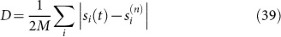between the evolved spin configuration and the learned spin configurations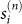. We see that the general behavior is that the time for the pattern completion scales as ~ 1/N, which can again be attributed to bosonic stimulated cooling. There is a logarithmic correction to this behavior, where there is an initial stiffness of the spins to move towards the steady state configuration. In Figure 3b we show the scaling of the time to reach a particular Hamming distancewith respect to N. We see that for large N all curves converge to the dominant ~ 1/N behaviour. This shows that BEC networks can equally well be used to perform tasks such as pattern completion, with the additional benefit of a reduced time in proportion to N.

## Discussion

We have analyzed the BEC network proposed in Ref. 6 in terms of the theory of neural networks and found that it is equivalent to a stochastic continuous Hopfield model. In contrast to the continuous Hopfield model where the overall timescale of the evolution is determined by the capacitance within each unit, in the BEC network the timescale is determined via the rate of cooling. Due to bosonic stimulated cooling, the rate of cooling may be accelerated in proportion to the number of bosons N on each site, which in turn accelerates the cooling rate of the entire system. The bosonic stimulated cooling makes the time evolution equations (29) on a single site not precisely the same as its Hopfield model counterpart (15), but the difference merely gives a modification of the dynamics as the system heads towards equilibrium, the overall behaviour of the system as a whole remains the same. In particular, tasks such as pattern completion may be performed using the BEC network, in the same way as the Hopfield model.

In this context, it would appear that using a BEC network, rather than a physical implementation of a Hopfield network, is nothing but a more complicated way of implementing what could be done equally well by either standard electronics or optical means12. Specifically, one could imagine using simply Hopfield circuits with small capacitances such that the timescale of the circuit is as small as desired. Other variations of optical implementations of Hopfield models allow for fast operation speeds. While for the zero temperature case this may be true, the BEC system does have the advantage that the random fluctuations following Boltzmann statistics is already built-in and do not require additional circuitry to simulate. Another possible advantage is that the speedups can be made systematically faster by simply increasing the number of bosons. At the level of the model that we present in this paper there is no fundamental limitation to the speedups that can be achieved. However, in practice other issues are likely to be present, so any speedup must be taken advantage of within the practical limitations that the experimental device imposes.

A possible issue for the physical realization is whether the connections between each Ising site require response times of the order of the cooling time on each site. Apart from a simple slowdown due to bottlenecks in the transmission, such delay times in the information between each site can introduce instabilities in the system causing divergent behavior. We leave as future work whether the proposals in Refs. 8,9,10 can be treated with the same analysis.

## Methods

First consider a single site and start with the probability distribution (18). Assuming that N 1, introduce the variables z = k/N,and the density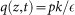of z at time t, the master equation is rewritten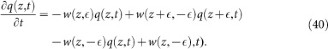Expanding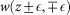and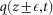up to second order in, we obtain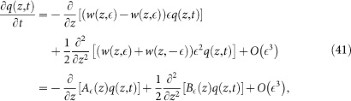where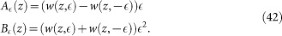Using the diffusion approximation such that the transition rates are on the order of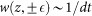,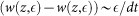and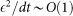and taking the limits of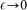, we obtain the Fokker-Planck equation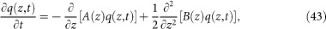where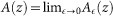and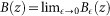. The corresponding stochastic differential equation is given by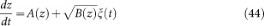where ξ(t) is Gaussian white noise with 〈ξ(t)〉 = 0 and 〈ξ(t)ξ(t′)〉 = δ(tt′). Changing variables to s = −1 + 2z, the coefficients for our case are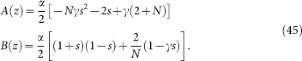The stochastic differential equation including noise is then obtained as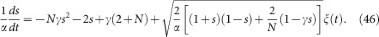A straightforward generalization to the multi-site case gives the following evolution equations